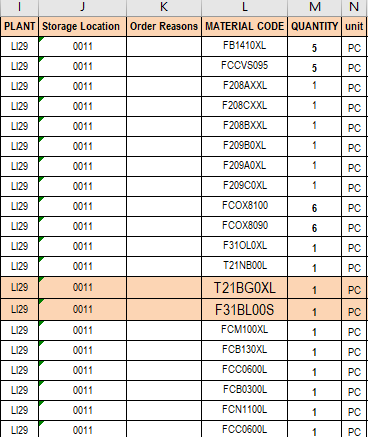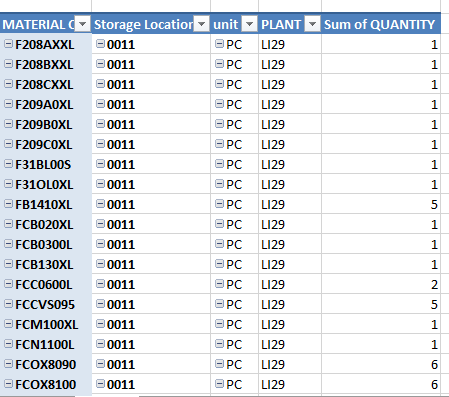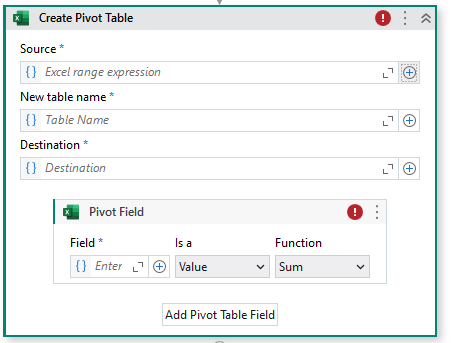# Pivot or Sumif In Datatable

Hi Team,
I have my Input as below,and my output is like below:This is like applying a pivot for the columns which can have duplicate material code but we need the output like for a group of specific plant, storage location, material code we need the sum of total quantity for that particular group.(Some thing like sumif in excel).

@yash.choursia Use Create Pivot table activityHi @drbuddepu i want to do it in datatable.

@yash.choursia

Create a outputdt with 5 columns and string type for each

Try this

`outputdt = (From d In dt.AsEnumerable Group d By k=d("PLANT").toString.Trim, k1=d("Storage Location").ToString.Trim, k2=d("Material Code").ToString.Trim,, k3=d("Unit").ToString.Trim Into grp = Group Let s1 = grp.Sum(function(x) CDBL(x("Quantity").ToString)).ToString Let ra = New Object(){k2,k1,k3,k,s1)} Select r = outputdt.Rows.Add(ra)).CopyToDataTable`

dt is the input datatable

Hope this helps

cheers

1 Like

Thanks @Anil_G . Means a lot. It worked.

1 Like

This topic was automatically closed 3 days after the last reply. New replies are no longer allowed.